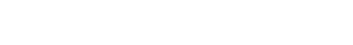Ranking loss被用在很多不同的领域，它有非常广泛应用，但缺乏命名标准化，导致了这个损失函数拥有很多其他别名，比如对比损失Contrastive loss，边缘损失Margin loss，铰链损失hinge loss和我们常见的三元组损失Triplet loss等。Ranking loss的目的是去预测输入样本之间的相对距离，这个任务经常也被称之为度量学习(metric learning)。

## 一、为什幺不用softmax？## 二、Triplet-loss原理

Triplet-loss的输入是一个三元组<a,p,n>

a：anchor

p：positive, 与 a 是同一类别的样本

n：negative, 与 a 是不同类别的样本easy triplets: L=0, 即d(a,p) + margin < d(a,n),这种情况不需要优化，天然(a,p)距离近，(a,n)距离远

hard triplets: d(a,n) < d(a,p),即(a,n)距离近,(a,p)距离远。

semi-hard triplets：d(a,p) < d(a,n) < d(a,p) + margin，即(a,n)的距离也很近，但相对与(a,p)会有一个margin，比easy triplets会稍微hard一些。

## 三、三元组选择方法

Easy negative example比较容易识别，因此没必要构建太多这个简单类型的三元组，否则会严重降低训练效率。若都采用hard类型，又可能会影响训练效果。这时，就需要一定的方法进行三元组的挑选，也就是“mine the triplets”。

### 3.2 Online mining — 在线挖掘

Online triplets mining的思想是为每一batch动态挖掘有用的三元组，即只计算batch中的triplets。将一个batch(B张图片)输入到神经网络中，得到B张图片的embedding，可组合出个三元组，但其中包含很多不可用的三元组(比如<a,p,p>或者<a,n,n>)。假设一个triplet<i,j,k>，如果i和j是同样的标签，i和k是不同的标签，这样的三元组才是可用三元组(valid triplets)。

Batch all：计算所有的valid triplet，对hard 和 semi-hard triplets上的loss进行平均(easy triplets不参与计算，平均会导致loss很小)，可以得到PK(K-1)(PK-K)个三元组。

Batch hard:对于每一个锚点，选择距离最大的正样本(a,p)和距离最小的负样本(a,n)，可以得到PK个三元组。

## 四、Triplet-loss在NLP领域中的应用

Triplet loss通常是在 个体级别的细粒度识别 上应用，比如精确到哪一个人的人脸识别，所以triplet loss的最主要应用也就是人脸识别face identification、跨境追踪技术person re-identification、车辆追踪vehicle re-identification等各种 identification 问题上。除了图片领域，NLP领域也有丰富的triplet-loss使用场景，比如推荐系统和文本匹配任务。在推荐系统中，候选集里的商品可能都是用户喜欢的，triplet-loss用于比较哪个商品对用户来说 更 适合。而在文本匹配任务中，对于锚点文本和一个待匹配的文本，如果用二分类问题是不合适的，可以用triplet-loss学出一个排序模型，输出的分数用于比较哪个更匹配。

2015年的IBM watson团队的的文章 Applying Deep Learning To Answer Selection: A Study And An Open Task ，损失函数也用到了triplet-loss。这篇论文的任务是问答，输入一个问题，从候选集中找到对应的回答。因此也可以看成释义识别任务，或者是短文本匹配。原文传送门 `https://arxiv.org/pdf/1508.01585.pdf`

## 五、Triplet-loss实践

### 5.1 数据准备### 5.2 建模

```def forward(self):
# Embedding Layer
a_embedding = self.dropout(tf.nn.embedding_lookup(self.embed, self.a))
p_embedding = self.dropout(tf.nn.embedding_lookup(self.embed, self.p))
n_embedding = self.dropout(tf.nn.embedding_lookup(self.embed, self.n))

# Representation Layer
with tf.variable_scope('a_lstm'):
self.a_output, self.a_state = self.LSTM(a_embedding)

with tf.variable_scope('p_lstm'):
self.p_output, self.p_state = self.LSTM(p_embedding)

with tf.variable_scope('n_lstm'):
self.n_output, self.n_state = self.LSTM(n_embedding)

# Cosine Layer
with tf.name_scope('cosine_similarity'):
self.cos_a_p = self.cal_cosine(self.a_state,self.p_state)
self.cos_a_n = self.cal_cosine(self.a_state,self.n_state)

# Loss（按照triplet loss的公式）
zero = tf.fill(tf.shape(self.cos_a_p),0.0)
margin = tf.fill(tf.shape(self.cos_a_n),args.margin)
losses = tf.maximum(zero,tf.subtract(margin,tf.subtract(self.cos_a_p,self.cos_a_n)))
self.loss = tf.reduce_sum(losses)

# 真实标签全为0，即【p,n】中，q和p为同一类,self.pred为输出的类别
self.y = tf.fill(tf.shape(self.cos_a_p),0)
expand_cos_ap = tf.expand_dims(self.cos_a_p,1)
expand_cos_an = tf.expand_dims(self.cos_a_n,1)
X = tf.concat([expand_cos_ap,expand_cos_an],1)
self.pred = tf.argmax(X,1)
correct_prediction = tf.equal(tf.cast(self.pred,tf.int32),self.y)
self.acc = tf.reduce_mean(tf.cast(correct_prediction,tf.float32),name="acc")```

### 5.3 效果测评

embedding_dim：300

lstm_hidden_size：100

batch_size：256

drop_out：0.9

epochs：15### 5.4 思考分析

cosine的相似度的取值范围是[-1,1]，在计算时应将其归一化到(0,1)的范围内，这样在计算loss时才能进行有效的反向传播。

## 六、参考文献

https://blog.csdn.net/u013066730/article/details/88797338

https://omoindrot.github.io/triplet-loss

https://www.jianshu.com/p/46c6f68264a1

https://zhuanlan.zhihu.com/p/136948465

https://www.jianshu.com/p/b1188c9f5fd2